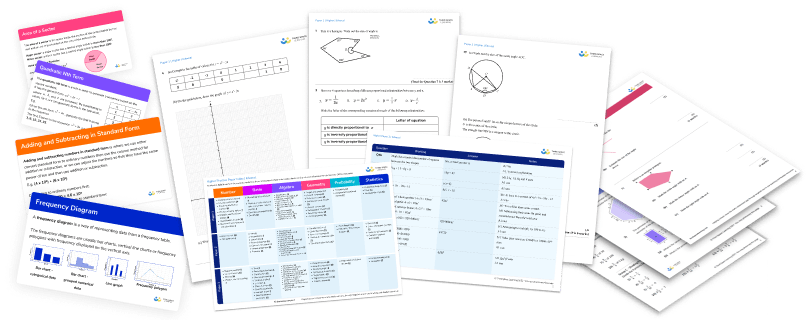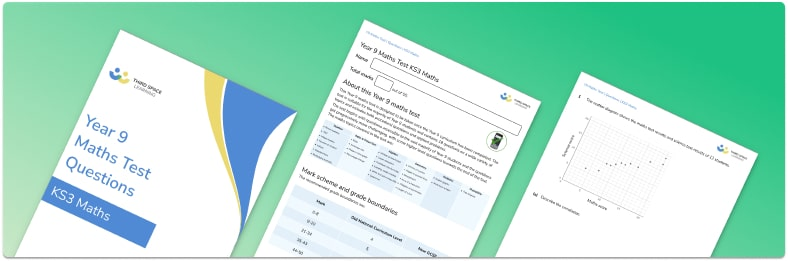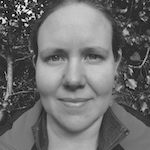Teaching support from the UK’s largest provider of in-school maths tuition.one to one lessonsschools supported

Scalable, affordable online support for KS3 students from maths and pedagogy experts

See it in action

FREE KS3 maths resources

Here you can find all of our free KS3 resources; explore the collections or navigate to each section to filter resources by topicExplore all resources
Key Stage 3

Scalable, affordable online support for KS3 students from maths and pedagogy experts

See it in action

# Free Year 9 Maths Test with Answers And Mark Scheme

This Year 9 maths test is perfect for assessing your students’ skills and maths knowledge. Including all the critical topics from the KS3 maths curriculum, this test is suitable for the majority of Year 9 students. The test includes both procedural questions and applied problems.

This test is designed to be taken at the end of year 9. If you’re looking for a test for the start of year 9, we recommend you download the free year 8 maths test instead as it will provide a much fairer assessment of what students know.

## What topics are likely to be covered in Year 9 maths

In Year 9 maths, students will build on all of the topics that are covered in Year 8. The topics cover a broad range of maths skills across:

• Number
• Algebra
• Ratio and proportion
• Geometry
• Statistics
• Probability

New topics that may be introduced for the first time in Year 9 include:

• Standard form
• Significant figures
• Direct and inverse proportion
• Pythagoras theorem
• Trigonometry
• Combined events probability
• Averages from a frequency table

You can check out our comprehensive revision guides and worksheets for the new topics covered in Year 9 here:Year 9 Maths Test

Download a free end of Year 9 maths test with 18 practice maths questions. Includes answers and mark scheme.Download FREE Now!

## Year 9 maths questions

Students in Year 9 are expected to answer a variety of questions. Some questions will be procedural and will focus on learning and practising new methods. Other questions will ask students to apply their knowledge to solve problems.

By Year 9 students should be comfortable with basic mental and written methods for calculating with integers, fractions, decimals and percentages. They should be familiar with applying these to a variety of multi-step problems and can be challenged to use these to solve problems that involve multiple topics.

Our Year 9 test contains 18 questions of increasing difficulty. The test begins with questions accessible to the vast majority of Year 9 students and the questions get progressively more challenging, with some higher level questions towards the end of the test. The maths topics covered in the test are:

The total number of marks available for the test is 50 and each question is worth between 1 and 3 marks. There are a range of question styles, including procedural questions, reasoning questions, multi-step applied questions and ‘show that’ questions. The mix of questions is similar to the mix that would be found in a GCSE maths exam paper.

## How to use this Year 9 maths test

• Use as an end of year test, once students have covered the KS3 curriculum.
• The test is suitable for the majority of Year 9 students and contains questions on a wide variety of topics and includes both procedural questions and applied problems.
• The test is one hour long, enabling it to be sat within a single lesson.
• Students may use a calculator.
• Encourage students to show all their working.
• Use the data collected on the attainment of your students to inform your next steps.

The summer term of Year 9 signals the end of KS3 and students’ transition to KS4 and GCSEs. The nature of maths is cumulative so if a child does not have a solid understanding of essential foundational concepts from KS3, they will struggle to keep up at KS4 and fall further behind.

End of year tests, such as ours, can offer a wealth of information for teachers, subject leads and SLT on the progress of a cohort. Use the data from this Year 9 test to identify topics for KS4 teachers to focus on and to identify groups and individual students who may need additional support.

You can also feedback to students individually or using whole class feedback so they know the areas they need to focus on as they move up the school.

Here at Third Space Learning, we provide personalised online one to one maths tuition for your in-need students, including those who are:

• At risk of not meeting expected standard
• Most impacted by the pandemic
• Lacking confidence or motivation to learn

We use diagnostic assessment to diagnose learning gaps and the misconceptions that are holding students back. With their dedicated tutor, students work through a personalised programme of lessons designed by curriculum experts to target their learning needs.

## Mark scheme and grade boundaries

There is a mark scheme provided with the test, including a breakdown of marks when a question is worth more than one mark.

## Year 9 maths test

Questions for our Year 9 maths test follow. Don’t forget to download the free Year 9 maths test for the answers and mark scheme to accompany these questions.

#### More support for Year 9

If there is a particular topic or area of study that you would like more ideas or support with, check out the GCSE maths revision pages from Third Space Learning, many of which are also relevant and useful to Year 9 maths students.

What maths should a Year 9 know?

By the end of Key Stage 3, students should have a broad understanding of many topics across the six main areas of maths:

Number: fractions, decimals, percentages, place value, negative numbers, factors and multiples, rounding, order of operations, standard form

Algebra: manipulating algebraic expressions, expanding and factorising, solving linear equations, using formulae, sequences, straight line graphs, inequalities

Ratio, proportion and rates of change: ratio, direct and inverse proportion, conversion rates

Geometry and measures: area and perimeter, surface area and volume of 3D shapes, angles, parallel lines, properties of polygons, transformations, speed/distance/time, construction and loci, bearings, congruence, Pythagoras theorem

Probability: theoretical probability, experimental probability, sample space diagrams, venn diagrams, probability of combined events

Statistics: data collection, pie charts, scatter diagrams, averages including from a table

You can find a full list of topics that students should cover by the end of KS3 here.

How do I prepare for Year 9?

Year 9 maths builds on the maths done in Years 7 and 8. You can prepare for Year 9 maths by revising topics covered in Years 7 and 8 and ensuring you are confident with as many of these as possible. Some good resources to help you with this are:

Revision guides available on Third Space Learning
Subscription sites that your school may use, for example mymaths or mathswatch
CGP workbooks or other maths revision guides/workbooks

What level is Year 9 maths?

By Year 9, there will be variation in the level that different students are working at. Most students in Year 9 will be working between GCSE grades 2 and 5. Different schools use different types of levels for Key Stage 3 maths so it is best to check how your school assigns levels to your work.

Year 9 work builds on work done in Years 7 and 8 and some new topics are introduced to prepare you for the GCSE course.

Do you have students who need extra support in maths?
Every week Third Space Learning’s maths specialist tutors support thousands of students across hundreds of schools with weekly online 1-to-1 lessons and maths interventions designed to plug gaps and boost progress.

Since 2013 we’ve helped over 150,000 primary and secondary students become more confident, able mathematicians. Find out more about our GCSE Maths tuition or request a personalised quote for your school to speak to us about your school’s needs and how we can help.

Personalised one to one maths tutoring for Year 7 students designed to help ease the transition to KS3.##### Beki Christian
Maths Teacher and Author
Beki has 11 years experience teaching secondary Maths. She is currently working as a Maths tutor as well as writing resources and blog posts for Third Space Learning.

Related Articles

x#### Year 9 Maths Test

Download a free end of Year 9 maths test with 18 practice maths questions. Includes answers and mark scheme.

#### Year 9 Maths TestDownload a free end of Year 9 maths test with 18 practice maths questions. Includes answers and mark scheme.